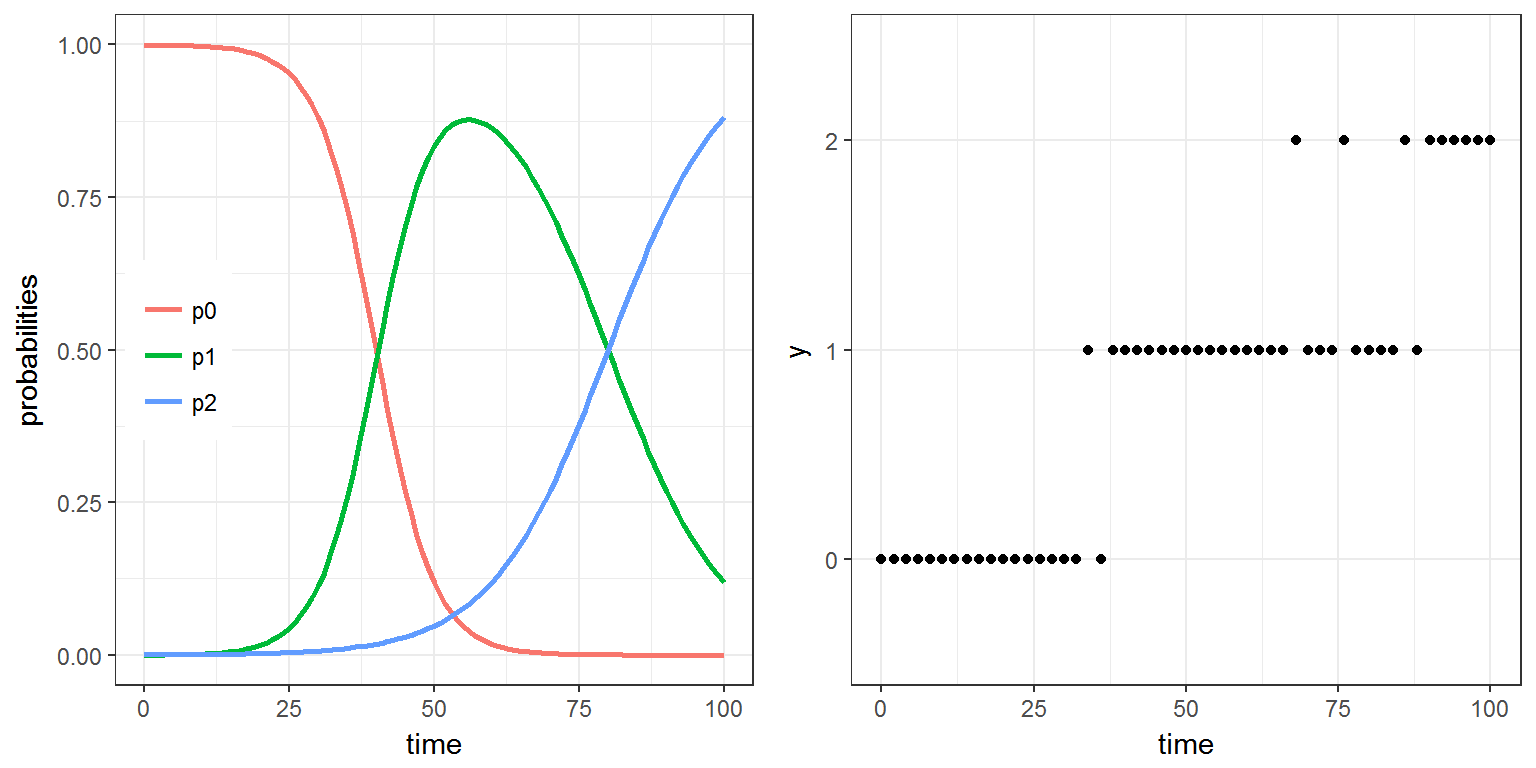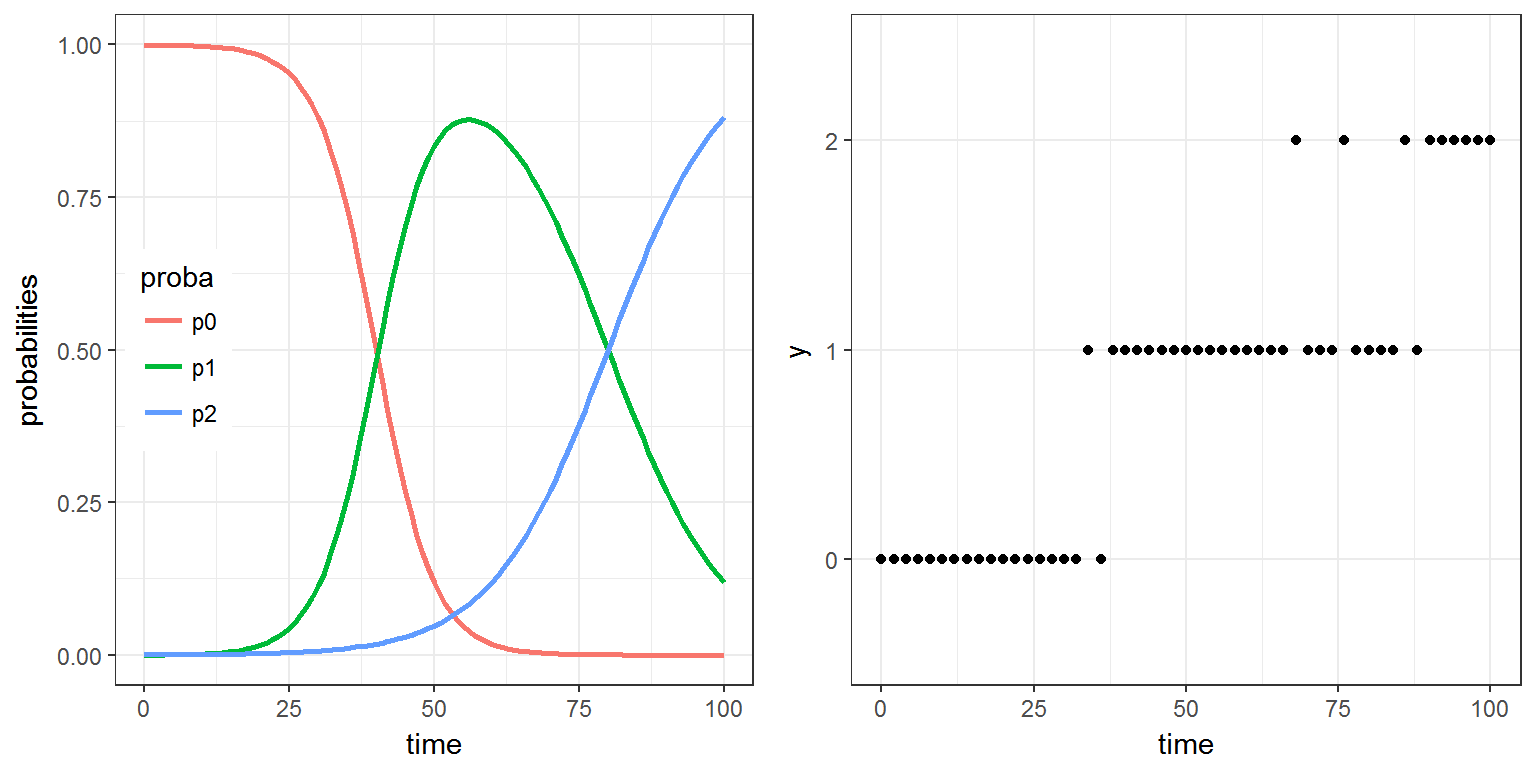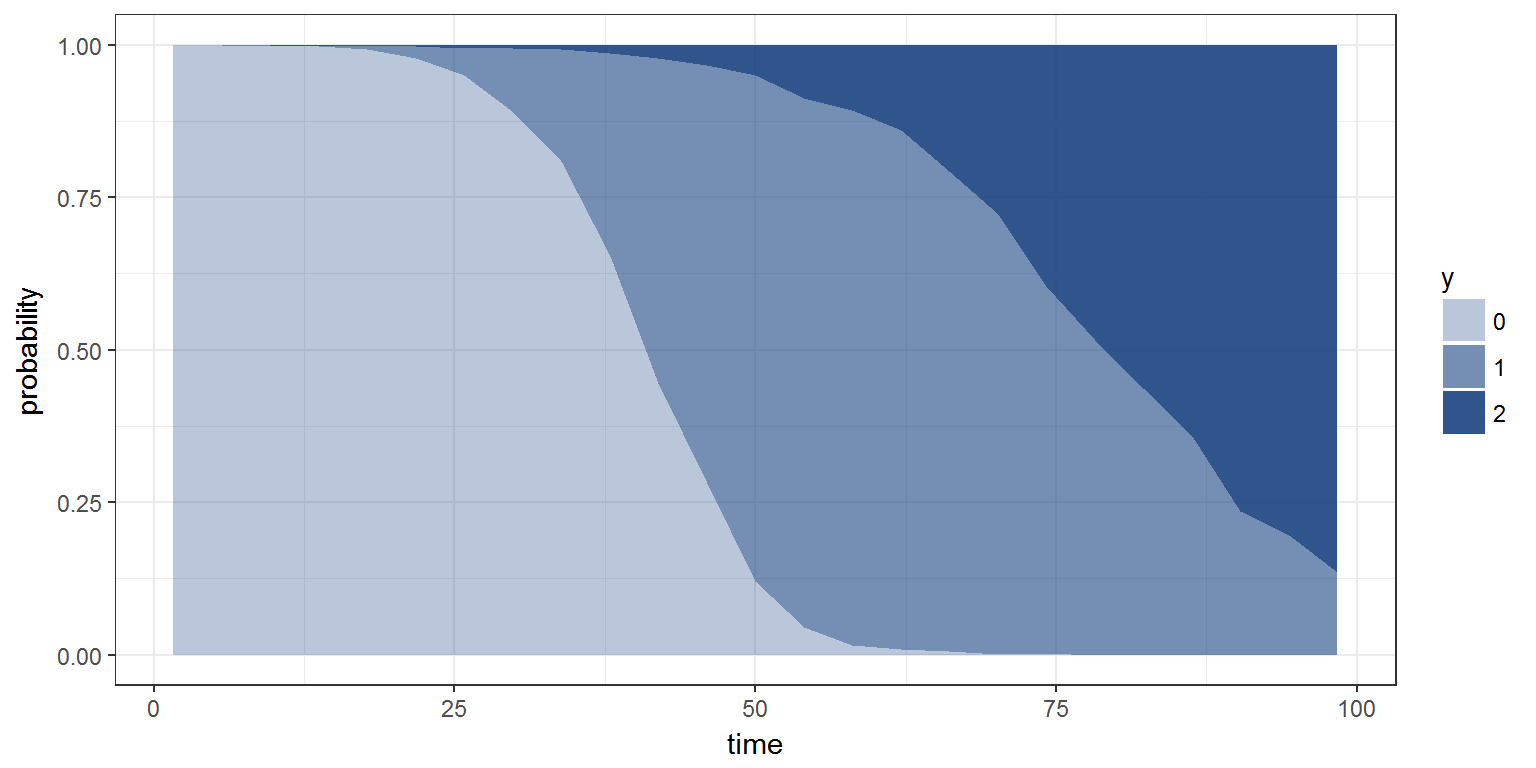R script: categorical.R

Mlxtran code: model/categoricalA.txt ; model/categoricalB.txt

$$\newcommand{\esp}{\mathbb{E}\left(#1\right)} \newcommand{\var}{\mbox{Var}\left(#1\right)} \newcommand{\deriv}{\dot{#1}(t)} \newcommand{\prob}{ \mathbb{P}\!(#1)} \newcommand{\eqdef}{\mathop{=}\limits^{\mathrm{def}}} \newcommand{\by}{\boldsymbol{y}} \newcommand{\bc}{\boldsymbol{c}} \newcommand{\bpsi}{\boldsymbol{\psi}} \def\pmacro{\texttt{p}} \def\like{{\cal L}} \def\llike{{\cal LL}} \def\logit{{\rm logit}} \def\probit{{\rm probit}} \def\one{{\rm 1\!I}} \def\iid{\mathop{\sim}_{\rm i.i.d.}} \def\simh0{\mathop{\sim}_{H_0}} \def\df{\texttt{df}} \def\res{e} \def\xomega{x} \newcommand{\argmin}{{\rm arg}\min_{#1}} \newcommand{\argmax}{{\rm arg}\max_{#1}} \newcommand{\Rset}{\mbox{\mathbb{R}}} \def\param{\theta} \def\setparam{\Theta} \def\xnew{x_{\rm new}} \def\fnew{f_{\rm new}} \def\ynew{y_{\rm new}} \def\nnew{n_{\rm new}} \def\enew{e_{\rm new}} \def\Xnew{X_{\rm new}} \def\hfnew{\widehat{\fnew}} \def\degree{m} \def\nbeta{d} \newcommand{\limite}{\mathop{\longrightarrow}\limits_{#1}} \def\ka{k{a}} \def\ska{k{\scriptscriptstyle a}} \def\kel{k{e}} \def\skel{k{\scriptscriptstyle e}} \def\cl{C{\small l}} \def\Tlag{T\hspace{-0.1em}{lag}} \def\sTlag{T\hspace{-0.07em}{\scriptscriptstyle lag}} \def\Tk{T\hspace{-0.1em}{k0}} \def\sTk{T\hspace{-0.07em}{\scriptscriptstyle k0}} \def\thalf{t{1/2}} \newcommand{\Dphi}{\partial_\pphi #1} \def\asigma{a} \def\pphi{\psi} \newcommand{\stheta}{{\theta^\star}} \newcommand{\htheta}{{\widehat{\theta}}}$$

# 1 Introduction

Assume now that the observed data takes its values in a fixed and finite set of nominal categories $$\{c_1, c_2,\ldots , c_K\}$$

Considering the observations $$(y_{j},\, 1 \leq j \leq n)$$ as a sequence of conditionally independent random variables, the model is completely defined by the probability mass functions $\prob{y_{j}=c_k} \quad \text{for} \ k=1,\ldots, K \ \text{and} \ 1 \leq j \leq n$

For a given $$j$$, the sum of the $$K$$ probabilities is 1, so in fact only $$K-1$$ of them need to be defined.

Ordinal data further assume that the categories are ordered: $c_1 \leq c_2,\leq \ldots \leq c_K .$

Instead of defining the probabilities of each category, it may be convenient to define the cumulative probabilities $\prob{y_{j} \leq c_k}, \quad \text{for} \ k=1,\ldots ,K-1$ or the cumulative logits ${\rm logit}\left(\prob{y_{j} \leq c_k}\right),\quad \text{for} \ k=1,\ldots ,K-1$

where $${\rm logit}(p) = \log(p/(1-p))$$.

The distribution of ordered categorical data can be defined in the block DEFINITIONof the Section [LONGITUDINAL]using either the probability mass functions, the cumulative probabilities or the cumulative logits.

# 2 Example

$$y_j$$ takes its values in $$\{0, 1, 2\}$$. We use cumulative logits to define the distribution of $$y_j$$:

\begin{align} {\rm logit}\left(\prob{y_{j} \leq 0}\right) &= a - b\, t_j \\ {\rm logit}\left(\prob{y_{j} \leq 1}\right) &= a - b\, t_j/2 \end{align} We can then derive the probabilities for each category: \begin{align} \prob{y_{j} = 0} &= \frac{1}{1+e^{-(a - b\, t_j)}} \\ \prob{y_{j} = 1} &= \frac{1}{1+e^{-(a - b\, t_j/2)}} - \prob{y_{j} = 0} \\ \prob{y_{j} = 2} &= 1 - \prob{y_{j} = 0} - \prob{y_{j} = 1} \end{align}

This model is implemented in the file categoricalA.txt:


[LONGITUDINAL]
input = {a,b}

EQUATION:
lp0 = a-b*t
lp1 = a-b*t/2
p0  = 1/(1+exp(-lp0))
p1  = 1/(1+exp(-lp1)) -p0
p2  = 1-p0-p1

DEFINITION:
y = {type       = categorical,
categories = {0, 1, 2},
P(y=0)     = p0,
P(y=1)     = p1}


We will use simulx for computing $$p_0$$, $$p_1$$ and $$p_2$$ every hour between 0h and 100h and for generating observations $$(y_j)$$ every 2 hours between 0h and 100h, with $$a=8$$ and $$b=0.2$$.

seed <- 12345
p    <- c(a=8,b=0.2)
pr   <- list(name=c('p0','p1','p2'), time=0:100)
y    <- list(name='y', time=seq(0, 100, by=2))
res  <- simulx(model     = 'model/categoricalA.txt',
parameter = p,
output    = list(pr, y),
settings  = list(seed=seed))

we can now plot the 3 probabilities and the simulated data:

library(gridExtra)
plot1=ggplot() + ylab("probabilities") +
geom_line(data=res$p0, aes(x=time, y=p0, colour="p0"),size=1) + geom_line(data=res$p1, aes(x=time, y=p1, colour="p1"),size=1) +
geom_line(data=res$p2, aes(x=time, y=p2, colour="p2"),size=1) + theme(legend.position=c(0.1,0.5),legend.title=element_blank()) plot2=ggplot() + geom_point(aes(x=time, y=y), data=res$y)
grid.arrange(plot1, plot2, ncol=2)We can equivalently use categoricalB.txt where the cumulative logits define the distribution of the $$y_j$$’s:


[LONGITUDINAL]
input =  {a,b}

EQUATION:
lp0 = a-b*t
lp1 = a-b*t/2

DEFINITION:
y = {type           = categorical,
categories     = {0,1,2},
logit(P(y<=0)) = lp0,
logit(P(y<=1)) = lp1}


We therefore use simulx for computing $$lp_0$$ and $$lp_1$$ and for generating observations $$(y_j)$$

lpr  <- list(name=c('lp0','lp1'), time=0:100)
res  <- simulx(model     = 'model/categoricalB.txt',
parameter = p,
output    = list(y,lpr),
settings  = list(seed=seed))

We can then derive the probability mass function from the cumulative logits

p0 <- 1/(1+exp(-res$lp0$lp0))
p1 <- 1/(1+exp(-res$lp1$lp1))-p0
p2 <- 1-p0-p1

and create a single data frame with these probabilities

library(reshape2)
pr <- data.frame(time=0:100,p0,p1,p2)
pr <- melt(pr ,  id = 'time', variable.name = 'proba')
# pr is a data frame with columns "id", "proba" and "value"
plot1=ggplot(pr, aes(time,value)) + geom_line(aes(colour = proba),size=1) +
ylab('probabilities') + theme(legend.position=c(.1, .5))
plot2=ggplot() + geom_point(aes(x=time, y=y), data=res$y) grid.arrange(plot1, plot2, ncol=2)When $$N$$ replicates of the data are simulated, catplotmlx can be used to display the empirical distribution of the simulated data: g <- list(size=1000) res <- simulx(model = 'model/categoricalA.txt', parameter = p, output = y, group = g) plot3 <- catplotmlx(res$y, breaks=25)
print(plot3)